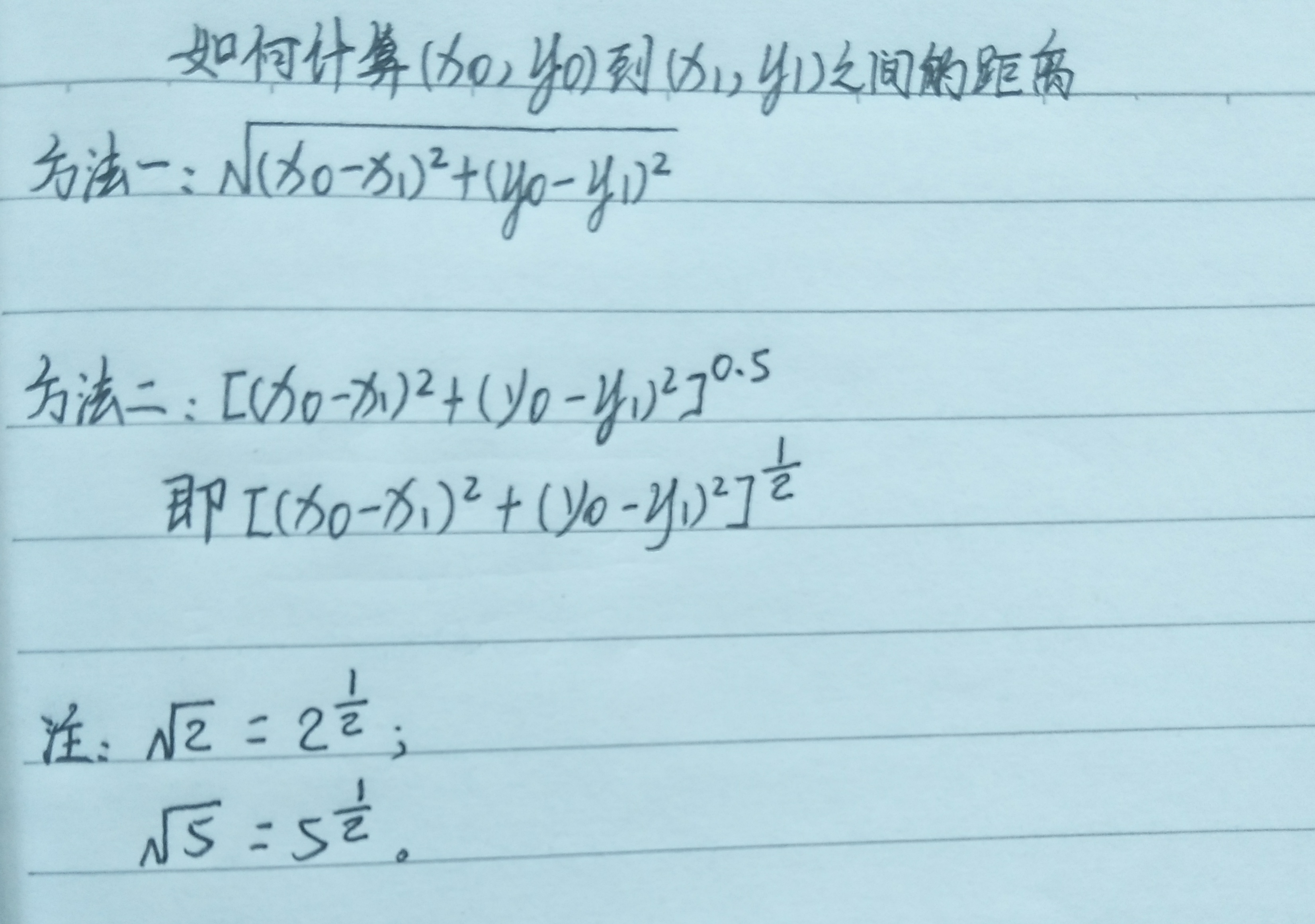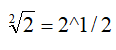• 今天小编就为大家分享一篇Python求两点之间的直线距离(2种实现方法)，具有很好的参考价值，希望对大家有所帮助。一起跟随小编过来看看吧
• import math class Point(): def __init__(self, x1, y1, x2, y2): self.x1 = x1 self.y1 = y1 self.x2 = x2 self.y2 = y2 class Line(Point): def __init__(self, x1,...
import math

class Point():
def __init__(self, x1, y1, x2, y2):
self.x1 = x1
self.y1 = y1
self.x2 = x2
self.y2 = y2

class Line(Point):
def __init__(self, x1, y1, x2, y2):
super().__init__(x1, y1, x2, y2)

def getlen(self):
changdu = math.sqrt(math.pow((self.x1 - self.x2), 2) + math.pow((self.y1 - self.y2), 2))
return changdu
L1 = Line(2,2,1,1)
print(L1.getlen())

展开全文• 求两点间距离 /***** 求两点间距离*****/ float getDistance(CvPoint pointO, CvPoint pointA) { float distance; distance = powf((pointO.x - pointA.x), 2) + powf((pointO.y - pointA.y), 2); distance = ...

求两点间距离
#***** 求两点间距离*****
def getDist_P2P(Point0,PointA):
distance=math.pow((Point0-PointA),2) + math.pow((Point0-PointA),2)
distance=math.sqrt(distance)
return distance


已知直线上两点求直线的一般式方程
常用的直线方程有一般式 点斜式 截距式 斜截式 两点式等等。除了一般式方程，它们要么不能支持所有情况下的直线（比如跟坐标轴垂直或者平行），要么不能支持所有情况下的点（比如x坐标相等，或者y坐标相等）。所以一般式方程在用计算机处理二维图形数据时特别有用。
已知直线上两点求直线的一般式方程
已知直线上的两点P1(X1,Y1) P2(X2,Y2)， P1 P2两点不重合。则直线的一般式方程AX+BY+C=0中，A B C分别等于：
A = Y2 - Y1
B = X1 - X2
C = X2*Y1 - X1*Y2

点到直线的距离:P到AB的距离

#***** 点到直线的距离:P到AB的距离*****
#P为线外一点，AB为线段两个端点
def getDist_P2L(PointP,Pointa,Pointb):
"""计算点到直线的距离
PointP：定点坐标
Pointa：直线a点坐标
Pointb：直线b点坐标
"""
#求直线方程
A=0
B=0
C=0
A=Pointa-Pointb
B=Pointb-Pointa
C=Pointa*Pointb-Pointa*Pointb
#代入点到直线距离公式
distance=0
distance=(A*PointP+B*PointP+C)/math.sqrt(A*A+B*B)

return distance


展开全文• 方法一： #导入math包 import math #定义的函数 class Point: def __init__(self,x=0,y=0): self.x=x self.y=y def getx(self): return self.x def gety(self): return self.y ...
 方法一：
#导入math包
import math
#定义点的函数
class Point:
def __init__(self,x=0,y=0):
self.x=x
self.y=y
def getx(self):
return self.x
def gety(self):
return self.y
#定义直线函数
class Getlen:
def __init__(self,p1,p2):
self.x=p1.getx()-p2.getx()
self.y=p1.gety()-p2.gety()
#用math.sqrt（）求平方根
self.len= math.sqrt((self.x**2)+(self.y**2))
#定义得到直线长度的函数
def getlen(self):
return self.len

#设置点p1的坐标为（0,0）
p1=Point(0,0)
#设置点p2的坐标为（3,4）
p2=Point(3,4)
#定义对象
l=Getlen(p1,p2)
#获取两点之间直线的长度
d=l.getlen())
方法二：
import numpy as np
import math
p1=np.array([0,0])
p2=np.array([1000,2000])
p3=p2-p1
p4=math.hypot(p3,p3)
print(p4)
博主技术交流Q群：1151493105
展开全文• ## 用Python如何计算两点间距离

万次阅读 多人点赞 2020-03-02 17:27:36
Python如何计算两点间距离？ 第一步，我们先来看一下两点间的距离公式。 两点间距离公式： ？？？这是个啥？ 是不是方法一还能看懂，方法二却一脸懵逼？ 请仔细看注释， 也就是说，根号2还可以写成2的...
                         用Python如何计算两点间距离？
第一步，我们先来看一下两点间的距离公式。
两点间距离公式：？？？这是个啥？是不是方法一还能看懂，方法二却一脸懵逼？
请仔细看注释，也就是说，根号2还可以写成2的0.5(也就是1/2)次方
这个是高中学过的知识，不知道你有没有想起来，哈哈......

第二步，我们需要学习一下pow()这个函数
pow(a,b)是计算a的b次方的一个函数
例如：pow(2,3)为2的3次方，为8

第三步，split()函数
split()函数是将数据按指定格式分割开，默认为空格
例1：
a = "0 1 3 5"
print(a.slpit())
将a中的数据以空格的分割开，并返回一个['0', '1', '3', '5']形式的列表
例2：
a = 0,1,3,5
print(s.split(","))
将a中的数据以逗号分割开，并返回一个['0', '1', '3', '5']形式的列表

第四步 format()格式化
format()格式化与%s, %d, %f有异曲同工之效，都是先为数据占一个位，而后补充数据
例1：
weekday = "星期一"
print('今天是%s' % weekday)
显示为：今天是星期一
例2：
weekday = "星期一"
print('今天是{}'.format(weekday))

第五步，我们看一下具体的代码~
从键盘上输入4个数字，各数字采用空格分隔，对应变量为x0, y0, x1, y1。计算两点(x0, y0)和(x1, y1)之间的距离。在屏幕上输出这个距离，保留2位小数。例如，键盘输入0 1 3 5，屏幕输出5.00

  #输入数据
ntxt=input('请输入4个数字(空格分隔):')
#利用split()函数，通过空格将数据分隔开并赋值给变量nls
nls=ntxt.split()
#利用eval()函数将字符串类型转化为数据类型；
#nls，将nls中的第零个数据赋值给变量x0
x0=eval(nls)
#nls，将nls中的第一个数据赋值给变量y0
y0=eval(nls)
#nls，将nls中的第二个数据赋值给变量x1
x1=eval(nls)
#nls，将nls中的第三个数据赋值给变量y1
y1=eval(nls)
#利用pow()函数计算两点间距离
r=pow(pow(x1-x0,2)+pow(y1-y0,2),0.5)
#打印数据；其中2f表示保留两位小数
print('{:.2f}'.format(r))
注：print('{:.2f}'.format(r))的意思是：用{:.2f}先占一个位置; .2f表示保留两位小数。
展开全文• 如图为一个10*10的网格图，黑点处为障碍物，给定任意两点——start和end，最短路径，走一格距离为1。 Code # 算法思想：通过BFS找end，找到就停 # 部分变量含义： # value->网格图列表 # hinders->障碍点...算法 广度优先
• 今天小编就为大家分享一篇python计算个地址之间距离方法，具有很好的参考价值，希望对大家有所帮助。一起跟随小编过来看看吧
• 任意维度两点间距离计算任意维坐标中两点之间距离:import math def distance(p0,p1,digits=2): a=map(lambda x: (x-x...
• 1013: 求两点间距离 时间限制: 1 Sec 内存限制: 30 MB 提交: 45272 解决: 27487 [状态] [讨论版] [提交] [命题人:admin] 题目描述 给定A(x1, y1), B(x2, y2)两点坐标，计算它们间的距离。 输入 输入包含四个实数x1, ...
• 今天小编就为大家分享一篇python实现个经纬度点之间距离和方位角的方法，具有很好的参考价值，希望对大家有所帮助。一起跟随小编过来看看吧
• 已知经纬度坐标求两点间距离，用python表示 已知地球上任意两点（lon1, lat1）,（ lon2, lat2）的经纬度坐标，求两点间的距离用haversine表示： 1.首先先将经纬度坐标的角度化成弧度（mlon1,mlat1）,(mlon2,mlat2) 2...
• 如下所示： distances = np.sqrt(np.sum(np.asarray(airportPosition - x_vals)**2, axis=1)) ... 您可能感兴趣的文章:python计算个地址之间距离方法Python实现的计算马氏距离算法示例Python实现计
• 主要为大家详细介绍了python广度优先搜索得到两点间最短路径，具有一定的参考价值，感兴趣的小伙伴们可以参考一下
• 可以任意输入两个空间点并求两点之间距离 如发现错误请给予指正 谢谢
• 种方法，一个是利用python库，另一个利用math函数计算，最后和百度地图测量的做对比 计算结果 447.249799354 446.72072701 447.02 百度地图测量 # 1. Python 库 from geopy.distance import geodesic aa = ...
• 主要介绍了Python实现的计算马氏距离算法,简单说明了马氏距离算法原理,并结合实例形式分析了Python实现与使用马氏距离算法的相关操作技巧,需要的朋友可以参考下
• 和平均距离(即对所有两点间距离求平均). 在20次实验完成后, 我们将根据20次实验得到的结果 计算圆上两点间最短距离,最长距离和平均距离的均值.   附: 在半径为15的圆上随机生成100个点坐标的代码...
• My question is there a function or library for python? 解决方案from math import * def greatCircleDistance((lat1, lon1), (lat2, lon2)): def haversin(x): return sin(x/2)**2 return 2 * asin(sqrt( ...
• 在处理GPS数据时，经常会给出两点的距离，来计算两点之间距离，这个距离大致可看作直线距离python版本代码如下所示： from math import radians, cos, sin, asin, sqrt def get_distance(lon1, lat1, lon2, ...
• 欧式距离（L2范数）是最易于理解的一种距离计算方法，源自欧式空间中两点间距离公式（如下图所示）。   #导入math包 import math #定义点的函数 class Point: def __init__(self,x=0,y=0): self.x=x self.y...
• 给定A(x1, y1), B(x2, y2)两点坐标，计算它们距离。 输入： 输入包含四个实数x1, y1, x2, y2，分别用空格隔开，含义如描述。其中0≤x1,x2,y1,y2≤100。 输出： 输出占一行，包含一个实数d，表示A, B...
• 4，write()方法写入计算的距离 示例代码如下，IDLE环境下可直接运行 import turtle import math x1,y1 = 100,100 x2,y2 = 100,-100 x3,y3 = -100,-100 x4,y4 = -100,100 turtle.penup() turt...海龟绘图 折线图
• 类似的需求应该比较多，这里的核心点是关于个坐标点之间距离的计算，之前没用过这个东西，刚好今天有个这样的需求，记录下。 import requests import psycopg2 import pandas as pd from math import radians, ...
• 本博文源于python基础，旨在探讨python中的lamda表达式应用。内容包含lamda格式/去除列表中偶数/扩大列表中的值/求两点坐标距离函数。列表 lambda
• from math import radians, cos, sin, asin, sqrt   def haversine(lon1, lat1, lon2, lat2): # 经度1，纬度1，经度2，纬度2   """  Calculate the great circle distance between two points  ......

# python求两点之间的距离python 订阅